# Fenwick Tree (Binary Indexed Tree)

### Why do we use the Fenwick tree?

Consider the following problem: we are given an array of integer values and asked to find the range sum between index [i, j]. Note that one-based indexing is used here. This can be done easily in O(j — i) time or O(n) time in the worst case using a single loop.

But now, suppose we have to find the range sum between any two indices multiple times. Then one basic idea would be to repeat the above process every time. If there are m range sum queries, then the worst time complexity would be m*O(n), which is O(mn). This is a brute force approach! Now the critical question is: can we think of some other efficient strategy to improve the time complexity further? Think!

If we know the prefix sum of the array till index 0 to i — 1 and index 0 to j, then we can easily calculate the sum between index [i, j]. How? Here is an idea: Sum[i, j] = PrefixSum[0, j] — PrefixSum[0, i — 1]. This calculation will take O(1) time if we know both values of the prefix sum. But the critical question is: how do we handle the situation of multiple range sum queries? Do we need to calculate the prefix sum every time? The answer is no! Here is a simple idea:

• We take an extra array PrefixSum[n] and do simple preprocessing to store the prefix sum of all indexes. We can easily construct the prefix sum array using a single loop in O(n) time.
• Now to find the sum between index [i, j] we can find PrefixSum[j] — PrefixSum[i — 1] and answer any query in O(1) time.

But here is another problem: suppose we want to change the value of some element in the original array. We can do this in O(1) time. But we will now need to reconstruct the prefix sum array again, which will take O(n) time.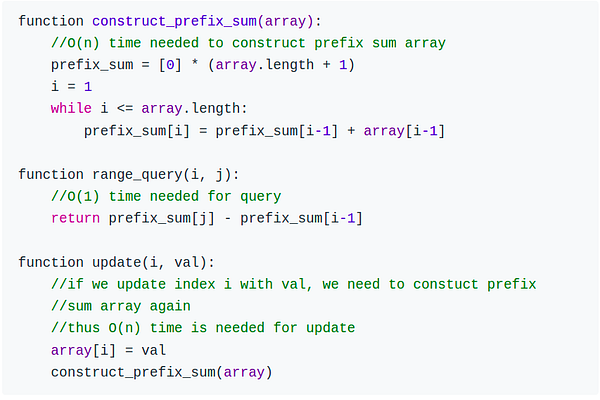Suppose, if there are m update queries and m range sum queries.

• The time complexity of update queries = m * time complexity of constructing prefix array = m * O(n) = O(mn).
• The time complexity of range sum queries = m * O(1) = O(m)

As we can see, there is a clear gap in the time complexity of both queries. If there are frequent update queries, this solution will take a lot of time. Now the critical question is: how do we handle this scenario and improve the time complexity of the update queries?

One solution to this problem is the idea of Fenwick Trees, which takes O(logn) time for both update and finding range sum. Definitely, this looks like a good tradeoff:

• Prefix array solution: Update query time complexity = O(n), Finding range sum time complexity = O(1)
• Fenwick tree solution: Update query time complexity = O(logn), Finding range sum time complexity = O(logn)

Let’s understand the idea and solution of the problem using the Fenwick tree. Fenwick tree was first described in the paper titled “A new data structure for cumulative frequency tables” (Peter M. Fenwick, 1994). Fenwick tree is also called Binary Indexed Tree(BIT).

### Fenwick Tree Problem Description

Let arr[] be an array of integers of length n and f is a sum function for finding the range sum between any two indexes l and r.

f(arr, l, r) = arr[l] + arr[l+1] + …… + arr[r].

Following are the specifications of the Fenwick tree data structure:

• Calculate the value of function f in the range [l, r] in O(log⁡n) time.
• Updates the value of an element of arr in O(log⁡n) time.
• Space complexity is O(n).

### What is Fenwick Tree?

The following image represents the Fenwick tree.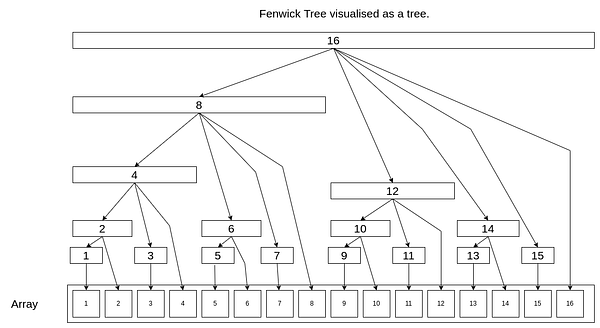A particular cell is also responsible for other cells in a Fenwick tree. The position of the first non-zero bit from right in the binary representation of the index of the cell determines the range of responsibility of that cell below itself. Let’s call this position RSB(rightmost set bit). Also, note that the positions from the right are one-based. The range of responsibility is 2^(RSB — 1).

This data structure is called a tree even though we store the data in an array as a single cell is responsible for the sum of multiple cells. The cell at index i is responsible for storing the sum of elements from (g(i), i).

• fTree[i] = sum(array[j]), where j is from g(i) to i.
• g(i) = 2^(RSB -1).

We can see that as the height of the tree is log(n), the update and query operations take log(n) time at max.

For example, the binary representation of 5 is 00101. So the range of responsibility will be 2^(1–1) = 1. For 12, the binary representation is 01100. So the range of responsibility will be 2^(3–1) = 4. The following table represents the range of responsibility for numbers from 1 to 16.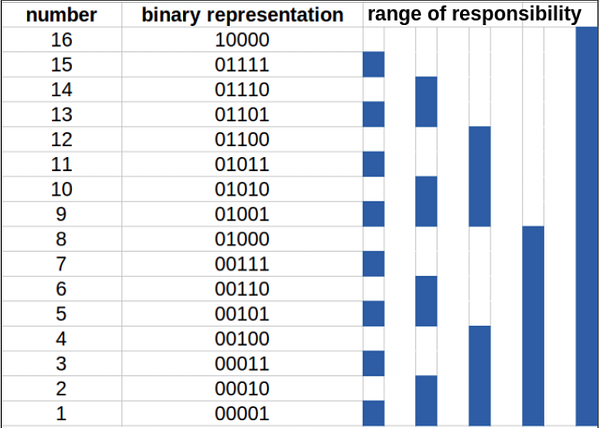The construction of the Fenwick tree is explained later. For now, suppose we have our Fenwick tree ready.

### How to handle range queries in Fenwick Tree?

We will first look at how to compute prefix sum up to a specific index which will eventually help us find range sum. Suppose you want to find prefix sum from 1 to i. Then you should start at index i and go downwards until you reach 0, adding the value at each index you land.

Now you want to find prefix sum up to index 5. Initialize answer with a tree and i with 5. Now subtract the current range of responsibility from i, which is 1. Therefore i = i -1 i.e. i = 4 now. Again add tree to the answer and subtract the current range of responsibility from i, which is 4. The index i becomes 0. So we have got prefix sum from [1,5] as our answer, which is a tree + tree in this case.

Similarly, do the same thing for finding prefix sum of [1, 15]. See the following table to understand how prefix sum up to 15 is calculated. The green regions are the ones that are used to find the answer.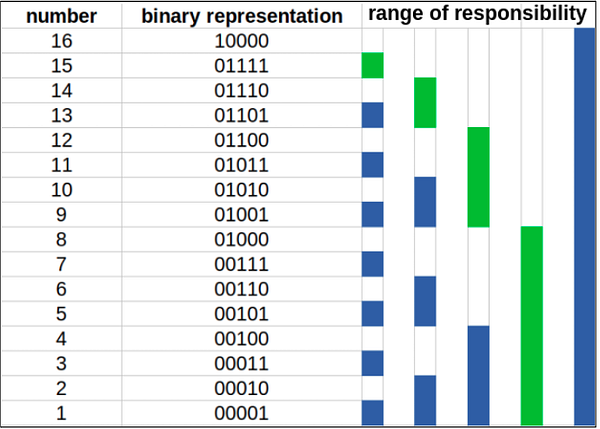Therefore prefixSum(15) = tree + tree + tree + tree.

We need a maximum log2(n)(as the maximum number of set bits can be log2(n)) operations to find the prefix sum. Thus the time complexity of range sum is O(logn). Now you can find range sum from index [i, j] by using rangeSum[i, j] = prefixSum[j] — prefixSum[i-1].

Pseudocode for range query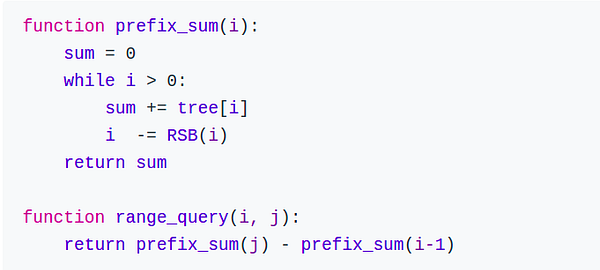Where RSB(i) returns the range of responsibility of i, RSB is implemented later.

### How to handle point updates in Fenwick Tree?

Point updates are the opposite of range queries. We will have to add the RSB to propagate the value upwards to the cells responsible for the current cell.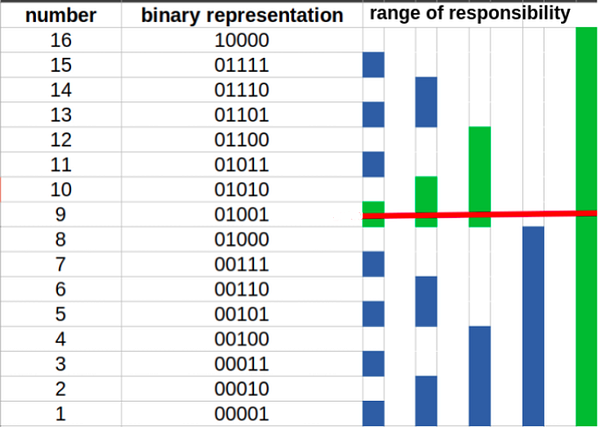As you can see from the above image, if we want to update the value at index 9 by increasing the value in the original array at index 9 by x, we would have to propagate upwards from 9 to 10, 10 to 12, and 12 to 16.

So the required updates are: tree += x, tree += x, tree += x, tree += x

Pseudocode for point update: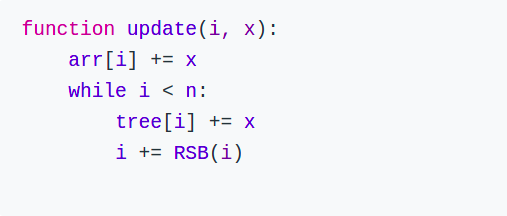### How to construct the Fenwick tree?

This is the first step that you have to do before answering any range sum or point update queries. You can create a tree with all values 0 initially and then do point updates for each index which will take O(nlogn) time, but we can do better.

We can construct the tree in linear time. The idea is to add the value in the current cell to the next immediate cell that is responsible for the current cell. It resembles the method for point updates but one cell at a time. This creates a cascading effect by propagating the value in each cell throughout the tree.

Let our current position be i. The immediate position responsible for i is i + RSB(i). We can consider cell i + RSB(i) as the parent of cell i. We must ignore this update if the parent cell is out of bounds.

Pseudocode for construction of Fenwick tree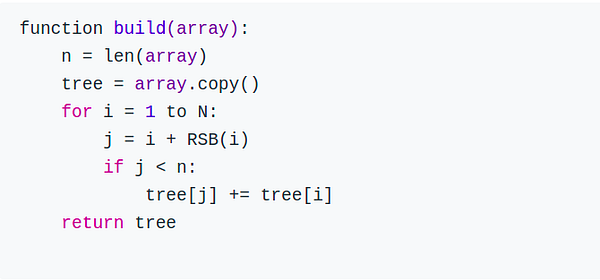### How does RSB work?

RSB of i is i & -i. It works because the negative of a number is produced by inverting the number, then adding 1 (that’s the definition of two’s complement). When you add 1, every bit starting at the bottom will overflow into the next higher bit; this stops once you reach zero. Those overflowed bits will all be zero, and the bits above the last one affected will be the inverse of each other, so the only bit left is the one that stopped the cascade — the one that started as 1 and was inverted to 0.

The above explanation was for range queries and point updates. We can also use the Fenwick tree for range updates and point queries and range updates and range queries. I will briefly explain the modifications we need to do for each of these cases.

The Fenwick tree is initialized with zeros. Suppose we want to increment the interval [l,r] by x. We need to make 2 point update operations on the Fenwick tree: add(l, x) and add(r+1, -x).

After these operations, the values from 0 to i < l have no effect. The values from l <= i <= r are incremented by x. And if i > r, then the second update operation will cancel the effect of the first one.

Thus, to get the value of arr[i], we take the prefix sum using the range sum method.

To handle both range updates and range queries, we require 2 Fenwick Trees, fTree1 and fTree2, initialized with zeros.

After a range update query, range_increment(l, r, delta), we would want the prefixSum(i) to have the following values depending on i:

1. i < l : prefixSum(i) = 0
2. l <= i <= r : prefixSum(i) = delta * (i-(l-1))
3. i > r : prefixSum(i) = delta * (r-(l+1))

which we can simplify as follows:

1. i < l : prefixSum(i) = 0 * i -0
2. l <= i <= r : prefixSum(i) = delta * i -delta * (l-1)
3. i > r : prefixSum(i) = 0 * i-(delta * (l-1)-delta * r)

Thus if we want to do a range update query, we can do it as follows: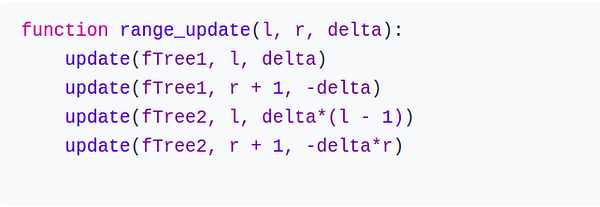and, prefixSum(i) = (prefixSum(fTree1, i) * i) - prefixSum(fTree2, i).

### Suggested Questions based on range sum query

Please write in the message below if you find anything incorrect, or you want to share more insight. Enjoy learning, Enjoy algorithms!